# Expanding Universe

The distant galaxies we see in all directions are moving away from the Earth, as evidenced by their red shifts. Hubble's law describes this expansion.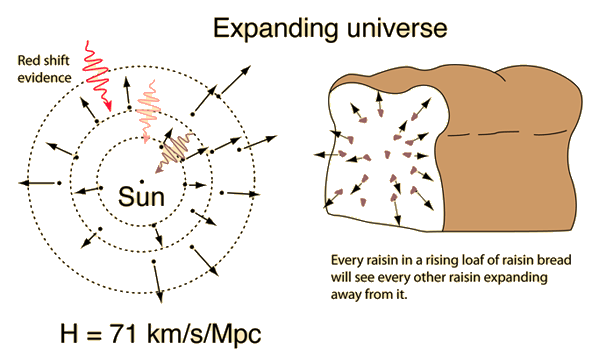The fact that we see other galaxies moving away from us does not imply that we are the center of the universe! All galaxies will see other galaxies moving away from them in an expanding universe unless the other galaxies are part of the same gravitationally bound group or cluster of galaxies. A rising loaf of raisin bread is a good visual model: each raisin will see all other raisins moving away from it as the loaf expands.

The fact that the universe is expanding then raises the question "Will it always expand?" Since the action of gravity works against the expansion, then if the density were large enough, the expansion would stop and the universe would collapse in a "big crunch". This is called a closed universe. If the density were small enough, the expansion would continue forever (an open universe). At a certain precise critical density, the universe would asymtotically approach zero expansion rate, but never collapse. Remarkably, all evidence indicates that the universe is very close to that critical density. Discussions about the expansion of the universe often refer to a density parameter Ω which is the density divided by the critical density, such that Ω = 1 represents the critical density condition.

 Newtonian model of expansion
 Calculation of expansion time
 How old is the universe?
 Physical keys to cosmology
Index

 HyperPhysics***** Astrophysics R Nave
Go Back

# Hubble's Law

Hubble's law is a statement of a direct correlation between the distance to a galaxy and its recessional velocity as determined by the red shift. It can be stated as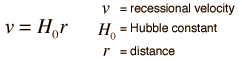The reported value of the Hubble parameter has varied widely over the years, testament to the difficulty of astronomical distance measurement. But with high precision experiments after 1990 the range of the reported values has narrowed greatly to values in the range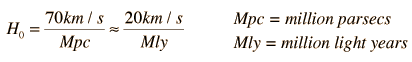An often mentioned problem for the Hubble law is Stefan's Quintet. Four of these five stars have similar red shifts but the fifth is quite different, and they appear to be interacting.

The Particle Data Group documents quote a "best modern value" of the Hubble parameter as 72 km/s per megaparsec (+/- 10%). This value comes from the use of type Ia supernovae (which give relative distances to about 5%) along with data from Cepheid variables gathered by the Hubble Space Telescope. The WMAP mission data leads to a Hubble constant of 71 +/- 5% km/s per megaparsec.

### Hubble distance calculation

 Calculation of expansion time
Index

Distance measurement

Distance unitsParticle Data Group

Section 18

 HyperPhysics***** Astrophysics R Nave
Go Back

# Hubble Parameter

The proportionality between recession velocity and distance in the Hubble Law is called the Hubble constant, or more appropriately the Hubble parameter we have a history of revising it. In recent years the value of the Hubble parameter has been considerably refined, and the current value given by the WMAP mission is 71 km/s per megaparsec.

The recession velocities of distant galaxies are known from the red shift, but the distances are much more uncertain. Distance measurement to nearby galaxies uses Cepheid variables as the main standard candle, but more distant galaxies must be examined to determine the Hubble constant since the direct Cepheid distances are all within the range of the gravitational pull of the local cluster. Use of the Hubble Space Telescope has permitted the detection of Cepheid variables in the Virgo cluster which have contributed to refinement of the distance scale.

The Particle Data Group documents quote a "best modern value" of the Hubble constant as 72 km/s per megaparsec (+/- 10%). This value comes from the use of type Ia supernovae (which give relative distances to about 5%) along with data from Cepheid variables gathered by the Hubble Space Telescope. The value from the WMAP survey is 71 km/s per megaparsec.

Another approach to the Hubble parameter gives emphasis to the fact that space itself is expanding, and at any given time can be described by a dimensionless scale factor R(t). The Hubble parameter is the ratio of the rate of change of the scale factor to the current value of the scale factor R: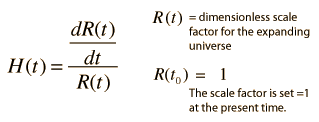The scale factor R for a given observed object in the expanding universe relative to R0 = 1 at the present time may be implied from the z parameter expression of the redshift. The Hubble parameter has the dimensions of inverse time, so a Hubble time tH may be obtained by inverting the present value of the Hubble parameter.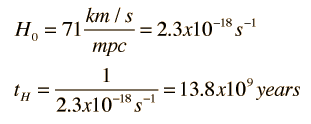One must use caution in interpreting this "Hubble time" since the relationship of the expansion time to the Hubble time is different for the radiation dominated era and the mass dominated era. Projections of the expansion time may be made from the expansion models.

### Hubble distance calculation

Index

Black hole concepts

 HyperPhysics***** Astrophysics R Nave
Go Back

# Hubble Parameter and Red Shifts

The Hubble Law states that the distance to a given galaxy is proportional to the recessional velocity as measured by the Doppler red shift. The red shift of the spectral lines is commonly expressed in terms of the z-parameter, which is the fractional shift in the spectral wavelength. The Hubble distance is given by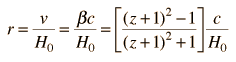and can be calculated from the wavelength shift of any spectral line. If a spectral line which is normally at nm is redshifted to nm, then z = and = v/c = .The Hubble distance is given by:

r = c/( km/s/Mpc) = Mpc = Mly

Carroll & Ostlie suggest that this distance relationship is not equivalent to the proper distance for large values of z, but that it differs from the proper distance by less than 5% for z<2.

Note: Values may be entered in any of the boxes to perform calculations. If needed parameters have not been entered, then they will default to values for the hydrogen red line with a 10% redshift and a Hubble constant of 70.

 Mpc = mega parsecs Mly = million light years
Index

Distance measurement

Reference:
Carroll & Ostlie
Sec 27.2

 HyperPhysics***** Astrophysics R Nave
Go Back